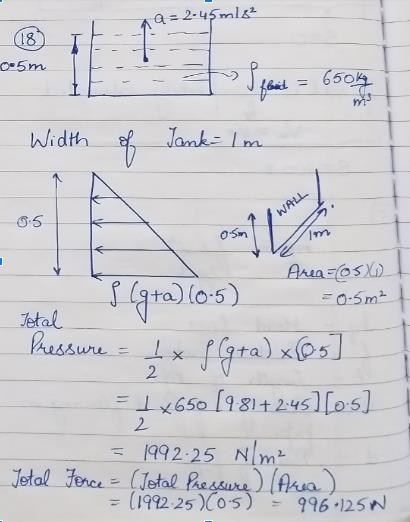Courses

# Test: Pressure Distribution in a Liquid

## 10 Questions MCQ Test Fluid Mechanics | Test: Pressure Distribution in a Liquid

Description
This mock test of Test: Pressure Distribution in a Liquid for Civil Engineering (CE) helps you for every Civil Engineering (CE) entrance exam. This contains 10 Multiple Choice Questions for Civil Engineering (CE) Test: Pressure Distribution in a Liquid (mcq) to study with solutions a complete question bank. The solved questions answers in this Test: Pressure Distribution in a Liquid quiz give you a good mix of easy questions and tough questions. Civil Engineering (CE) students definitely take this Test: Pressure Distribution in a Liquid exercise for a better result in the exam. You can find other Test: Pressure Distribution in a Liquid extra questions, long questions & short questions for Civil Engineering (CE) on EduRev as well by searching above.
QUESTION: 1

### A rectangular tank is moving horizontally in the direction of its length with a constant acceleration of 3.6 m/s2. If tank is open at the top then calculate the angle of water surface to the horizontal.

Solution:

Explanation: tanθ=a/g
tanθ=3.6/9.8
θ=20.15⁰.

QUESTION: 2

### A rectangular tank is moving horizontally in the direction of its length with a constant acceleration of 4.8 m/s2. The length of tank is 7 m and depth is 1.5 m. If tank is open at the top then calculate the maximum pressure intensity at the bottom.

Solution:

Explanation: tanθ=a/g
tanθ=4.8/9.8
θ=26.07⁰
h= d+(L/2)tanθ
= 1.5+3.5tan26.07
= 3.21 m
p=ρ*g*h
=3.15 N/cm2.

QUESTION: 3

### A rectangular tank is moving horizontally in the direction of its length with a constant acceleration of 5.5 m/s2. The length of tank is 5.5 m and depth is 2 m. If tank is open at the top then calculate the minimum pressure intensity at the bottom.

Solution:

Explanation: tanθ=a/g
tanθ=5.5/9.8
θ=29.28⁰
h= d-(L/2)tanθ
= 2-2.75 tan29.28
= 3.21 m

p=ρ*g*h
=1.9 N/cm2.

QUESTION: 4

A rectangular tank is moving horizontally in the direction of its length with a constant acceleration of 4.5 m/s2.The length, width and depth of tank are 7 m, 3m, 2.5m respectively. If tank is open at the top then calculate the total force due to water acting on higher pressure end of the tank.

Solution:

Explanation: tanθ=a/g
tanθ=4.5/9.8
θ=24.64⁰
h= d+(L/2)tanθ
= 2.5+3.5tan24.64
= 4.1 m

F=wAĥ
=9810*2.68*4.1
= 1.07 MN.

QUESTION: 5

A tank containing water upto a depth of 500 mm is moving vertically upward with a constant acceleration of 2.45 m/s2. Find the force exerted by fluid of specific gravity .65 on the side of tank,width of tank is 1m.

Solution:QUESTION: 6

A tank containing water upto a depth of 750 mm is moving vertically downward with a constant acceleration of 3.45 m/s2. Find the force exerted by fluid of specific gravity .85 on the side of tank,width of tank is 2m

Solution:

Explanation: p=ρ*g*h*(1-a/g)
=750*9.81*0.75*(1-3.45/9.81)
=3577 N/m2
F=wAĥ
= 0.5*0.75*3984.5*2
= 2682.75 N.

QUESTION: 7

A tank containing water upto a depth of 650 mm is stationary. Find the force exerted by fluid of specific gravity .55 on the side of tank,width of tank is 1.5m

Solution:

Explanation: p=ρ*g*h
=550*9.81*0.65
=3507 N/m2
F=wAĥ
= 0.5*0.65*3507*1.5
= 1709.7N.

QUESTION: 8

The pressure intensity at the bottom remains same, even if the tank moves with constant horizontal acceleration.

Solution:

Explanation: The pressure intensity at the bottom differs due to variation in height as tank moves with constant acceleration.

QUESTION: 9

There will be development of shear stress due to the dynamic motion of tank or container.

Solution:

Explanation: The water in tank is at rest even if the tank is moving.

QUESTION: 10

If the tank is moving vertically, which of its component is subjected to maximum total pressure?

Solution:

Explanation: Base bores the direct pressure.